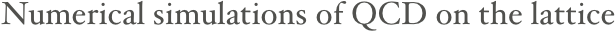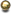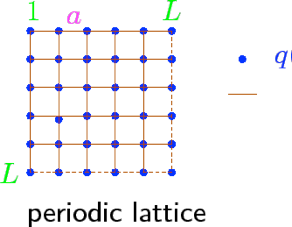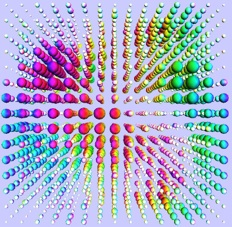Analytic or perturbative solutions in low-energy QCD are hard or impossible due to the highly nonlinear nature of the strong force. Lattice QCD is a well-established non-perturbative approach to solving QCD. It is formulated on a grid or lattice of points in space and time. This formulation of QCD in discrete rather than continuous spacetime naturally introduces a momentum cut off at the order 1/a, where a is the lattice spacing, which regularizes the theory. As a result, lattice QCD is mathematically well-defined. Most importantly, lattice QCD provides a framework for investigation of non-perturbative phenomena such as confinement and Quark-Gluon Plasma formation, which are intractable by means of analytic field theories.

In lattice QCD, fields representing quarks are defined at lattice sites, while the gluon fields are defined on the links connecting neighboring sites. This approximation approaches continuum QCD as the spacing between lattice sites is reduced to zero. Because the computational cost of numerical simulations can increase dramatically as the lattice spacing decreases, results are often extrapolated to a=0 by repeated calculations at different lattice spacings a that are large enough to be tractable.

Numerical lattice QCD calculations using Monte Carlo methods can be extremely computationally intensive, requiring the use of the largest available supercomputers.

Research topics include:

1.QCD equation of state with 2+1 and 2+1+1 dynamical flavors

1.study of chiral and deconfinement phase transitions and determination of

the QCD transition temperature

1.diagonal and non-diagonal quark number susceptibilities

1.in-medium spectral functions of light and heavy qqbar pairs

1.Hadron Resonance Gas model and its interpretation of the lattice data

OverviewCollaborations

C. Ratti is a member of the Wuppertal-Budapest collaboration and is actively working with the group of Prof. Zoltan Fodor at Wuppertal University